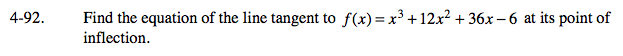### Home > CALC > Chapter 4 > Lesson 4.2.4 > Problem4-92

4-92.Find the inflection point of a cubic function can be found by setting f ''(x) = 0 or f ''(x) = DNE. What is the slope at that point?
What is the y-value at that point?

(show work)
y = −12x − 70